### OpenVINO™ 手写字符识别模型与使用openlab_4276841a 更新于 10月前

#### 引言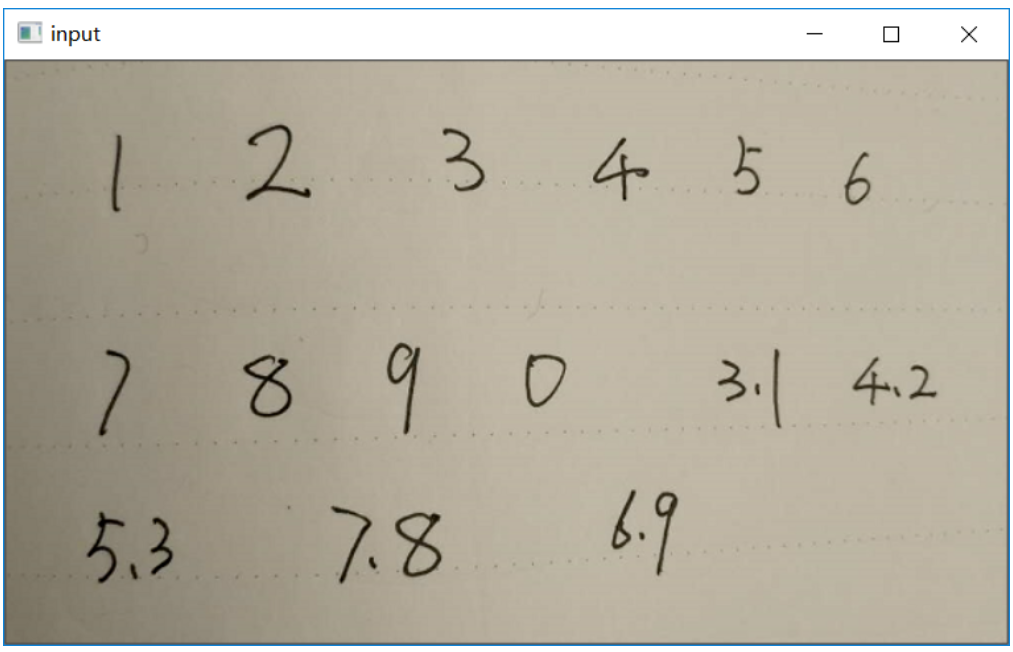#### 模型使用与演示

Step 1: 读取图象并二值化

``Mat src = imread("D:/images/zsxq/ocr.png");imshow("input", src);Mat gray, binary;cvtColor(src, gray, COLOR_BGR2GRAY);adaptiveThreshold(gray, binary, 255, ADAPTIVE_THRESH_GAUSSIAN_C, THRESH_BINARY_INV, 25, 10);``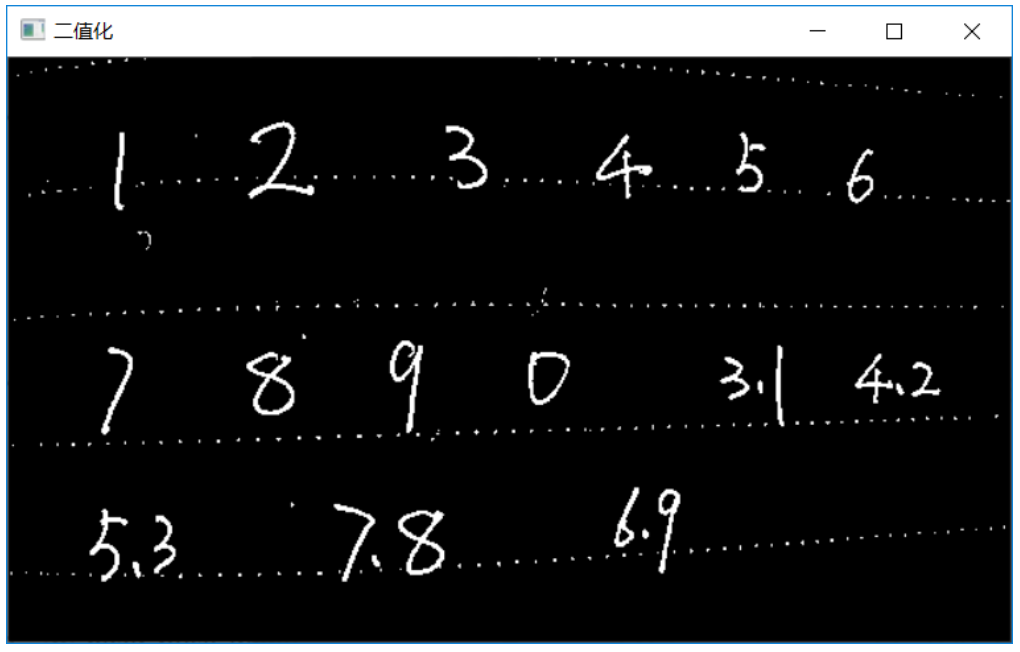图-2（自适应二值化）

Step 2: 使用轮廓分析过填充过滤小噪点

``std::vector<vector<Point>> contour****r>std::vector<Vec4i> hireachy;findContour***inary, contours, hireachy, RETR_EXTERNAL, CHAIN_APPROX_SIMPLE);int image_height = src.row****r>int image_width = src.col****r>for (size_t t = 0; t < contours.size(); t++) {double area = contourArea(contours[t]);if (area < 10) {drawContour***inary, contours, t, Scalar(0), -1, 8);}}``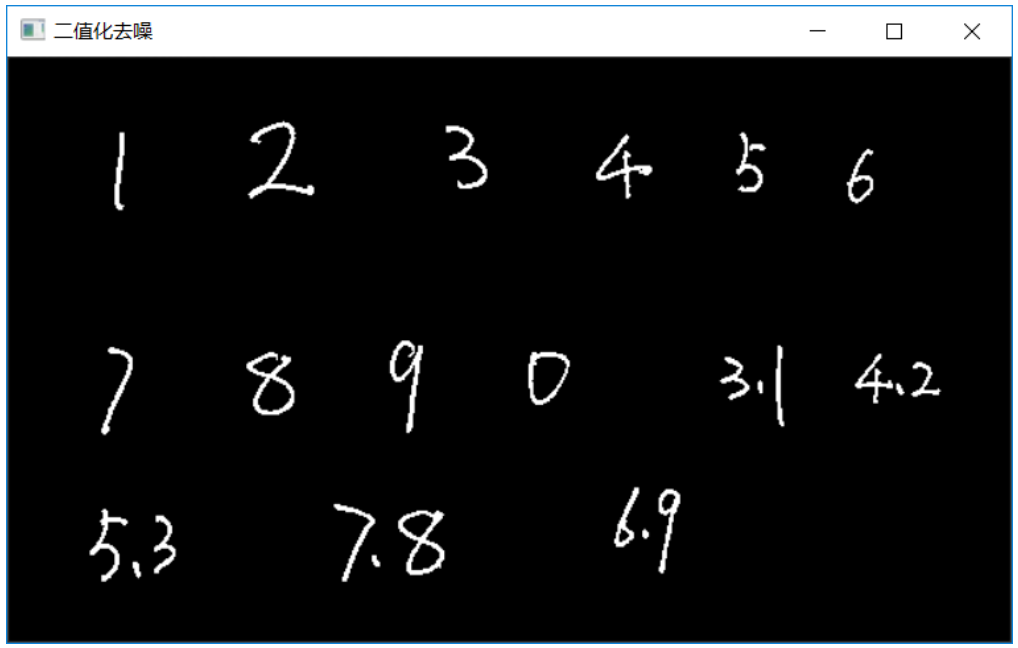Step 3：膨胀预处理

``Mat se = getStructuringElement(MORPH_RECT, Size(45, 5));Mat temp;dilate(binary, temp, se);``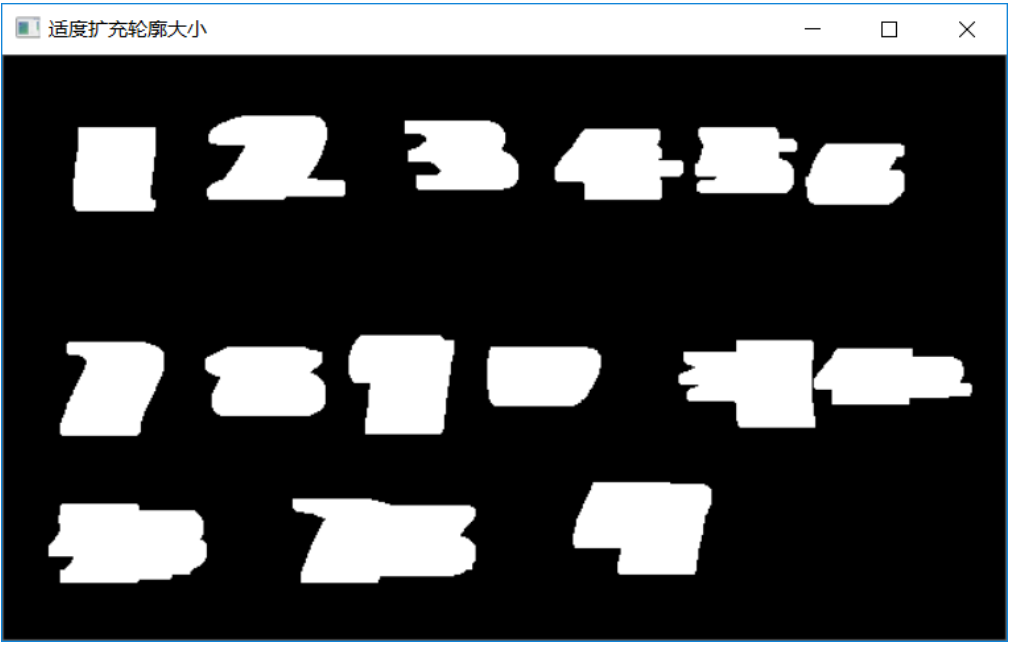Step 4：数字识别推理与解析

``// 处理输出结果findContours(temp, contours, hireachy, RETR_EXTERNAL, CHAIN_APPROX_SIMPLE);for (size_t t = 0; t < contours.size(); t++) {Rect box = boundingRect(contours[t]);Mat roi = gray(box);size_t image_size = h*w;Mat blob_image;resize(roi, blob_image, Size(w, h));// NCHWunsigned char* data = static_cast<unsigned char*>(input->buffer());for (size_t row = 0; row < h; row++) {for (size_t col = 0; col < w; col++) {data[row*w + col] = blob_image.at<uchar>(row, col);}}// 执行预测infer_request.Infer();auto output = infer_request.GetBlob(output_name);const float* blob_out = static_cast<PrecisionTrait<Precision::FP32>::value_type*>(output->buffer());const SizeVector reco_dims = output->getTensorDesc().getDim******r>const int RW = reco_dims;const int RB = reco_dims;const int RL = reco_dims;std::string ocr_txt = ctc_decode(blob_out, RW, RL);std::cout << ocr_txt << std::endl;cv::putText(src, ocr_txt, box.tl(), cv::FONT_HERSHEY_PLAIN, 1.0, cv::Scalar(255, 0, 0), 1);cv::rectangle(src, box, Scalar(0, 0, 255), 2, 8, 0);}``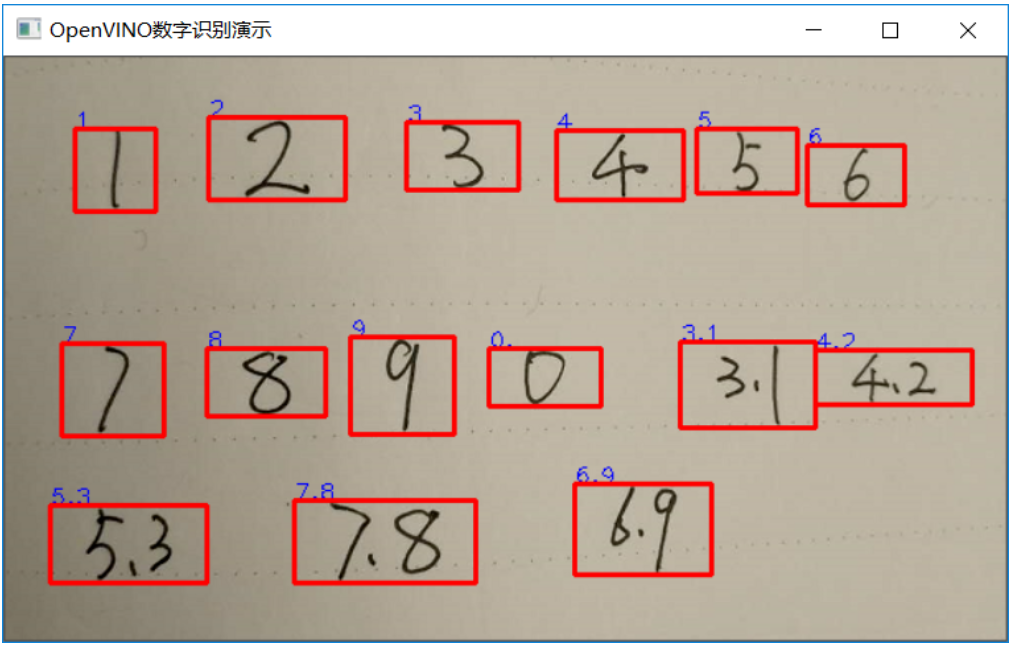#### 扩展探索

``Rect box = boundingRect(contours[t]);float rate = box.width / box.height;if (rate > 1.5) {continue;}box.x = box.x - 15;box.width = box.width + 30;box.y = box.y - 5;box.height = box.height + 10;``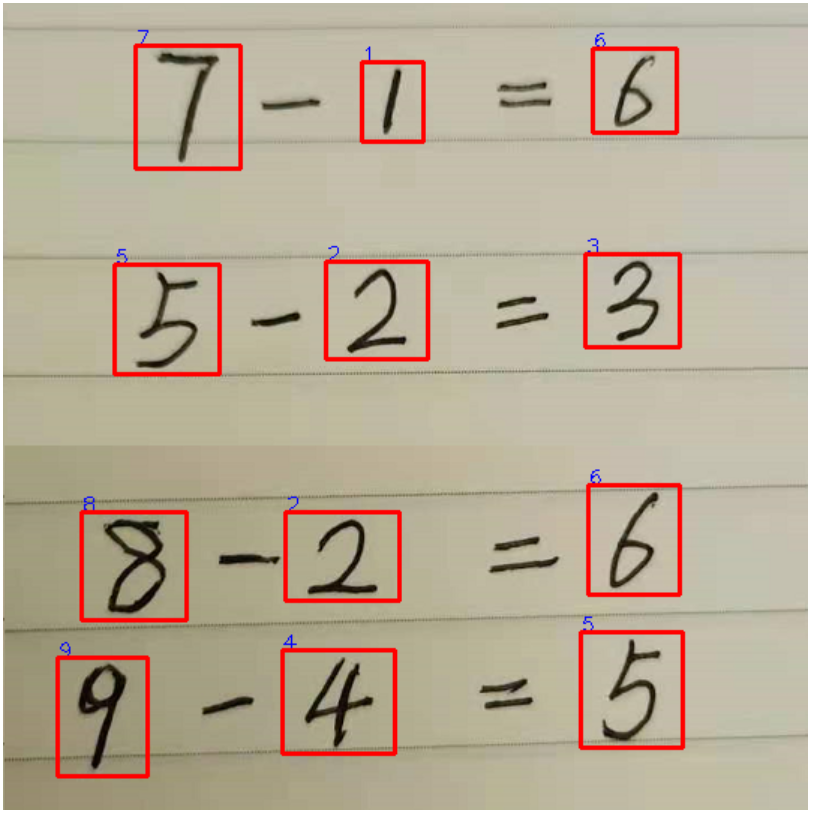图-6

0个评论